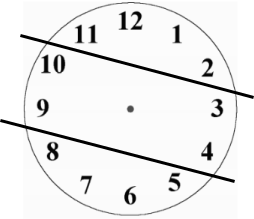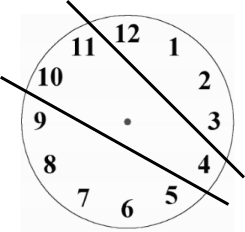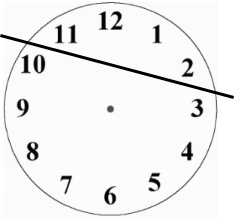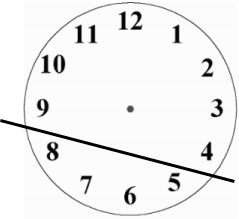#### You may also like### Consecutive Numbers

An investigation involving adding and subtracting sets of consecutive numbers. Lots to find out, lots to explore.### Have You Got It?

Can you explain the strategy for winning this game with any target?### Counting Factors

Is there an efficient way to work out how many factors a large number has?

# Split Clock Face

##### Age 11 to 14 ShortChallenge Level
Answer:Each sum equal to $\frac{1}{3}$ of $1 + 2 + 3 + 4 + 5 + 6 + 7 + 8 + 9 + 10 + 11 + 12$$=\frac13$ of $78=26$

2 of the 3 parts will consist only of numbers that are next to each other on the clock face, as shown on the right

Finding numbers that work
12 with 1                       or            12 not with 1
12 + 1 = 13                                  12 + 11 = 22
12 + 1 + 2 = 15                            12 + 11 + 10 = 32 too big
11 + 12 + 1 + 2 = 2610 + 3 = 13
10 + 3 + 9 = 22
10 + 3 + 9 + 4 = 26check: 5 + 6 + 7 + 8 = 26 too.

Using algebra
Of the two sections of touching numbers on the clock face that add up to 26, at most one of them will contain the 12 and the 1. So at least one of them will not contain the 12 and the 1, so at least one of them will be a set of ascending numbers (that go up by 1 each time).

If the smallest of the numbers is $n$, then the next one will be $n+1$, the next one will be $n+2$ and so on. So we need either $n+(n+1)=26$, or $n+(n+1)+(n+2)=26$, or $n+(n+1)+(n+2)+(n+3)=26$, and so on.

If $n+(n+1)=26$, then $2n+1=26$, so $2n=25$ so $n=12.5$, which is not a number on the clock face.

If $n+(n+1)+(n+2)=26$, then $3n+3=26$, so $3n=23$ so $n=7.\dot{6}$, which is not a number on the clock face.

If $n+(n+1)+(n+2)+(n+3)=26$, then $4n+6=26$, so $4n=20$ so $n=5$. So $5 + 6 + 7 + 8 = 26$, and so one of the lines could go here.Now choosing where the other line might go, note that $9+10=19<26$ but $9+10+11=30>26$, so 9 and 10 must go with some numbers from the right hand side of the clock face. $9+10+4=23<26$ but $9+10+4+3=26$.

That would leave $11+12+1+2=26$ as well. So the clock face can be split up as shown below.Note: this method gives us a way to check that there is no other way to do this.

If $n+(n+1)+(n+2)+(n+3)=26$, then $n=5$, and we have one way to split up the clock face.

If $n+(n+1)+(n+2)+(n+3)+(n+4)=26$, then $5n+10=26$, so $5n=16$ so $n=3.2$, which is not a number on the clock face.

If $n+(n+1)+(n+2)+(n+3)+(n+4)+(n+5)=26$, then $6n+15=26$, so $6n=11$ so $n=1.8\dot{3}$, which is not a number on the clock face.

If $n+(n+1)+(n+2)+(n+3)+(n+4)+(n+5)+(n+6)=26$, then $7n+21=26$, so $7n=5$ so $n=\frac{5}{7}<1$. There are no numbers less than 1 on the clock face, so, since adding more numbers will only make $n$ smaller, this will not work for any other sums of consective numbers.

You can find more short problems, arranged by curriculum topic, in our short problems collection.